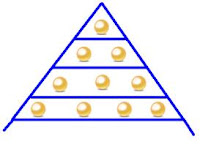## Wednesday, 27 February 2013

### CBSE Class 10 - Maths - Arithmetic Progression (MCQs)

Arithmetic Progression

MCQs

Q1: In an AP, if d = -4, n=7, an = 4, then a is

(a) 6
(b) 7
(c) 20
(d) 28

Q2(CBSE): Which term of the A.P. 24, 21, 18, ................... is the first negative term?

(a) 8th
(b) 9th
(c) 10th
(d) 12th

Q3(SQP): The sum of first five multiples of 3 is:

(a) 45
(b) 65
(c) 75
(d) 90

Q4(CBSE): For an A.P. if a25 – a20 = 45, then d equals to:

(a) 9
(b) –9
(c) 18
(d) 23

Q5(CBSE):  If a + 1, 2a + 1, 4a – 1 are in A.P., then the value of a is:

(a) 1
(b) 2
(c) 3
(d) 4

Q6(CBSE): 15th term of the A.P., x – 7, x – 2, x + 3,.... is

(a) x + 63
(b) x + 73
(c) x + 83
(d) x + 53

Q7: (1) + (1 + 1) + (1 + 1 + 1) + ... + (1 + 1 + 1 + ... n - 1 times) = ....

(a) n(n+1)/2
(b) (n-1)n/2
(c) n2
(d) n

Q8: For AP. T18 - T8 = ..... .

(a) d
(b) 10d
(c) 26d
(d) 2d

Q9(CBSE): Which term of the A.P. 92, 88, 84, 80, .... is 0?

(a) 23
(b) 32
(c) 22
(d) 24

Q10: If an A.P. has a = 1, tn = 20 and Sn = 399, then value of n is :

(a) 20
(b) 32
(c) 38
(d) 40

1:(d) 28
[Soln: an = a + (n - 1)× d
⇒ 4 = a + (7 - 1) × -4
⇒ 4 = a - 24
⇒ a = 4 + 24 = 28]

2: (c) 10th
[Soln: an = a + (n - 1)× d where d = -3, Let an = 0
⇒ 0 = 24 + (n - 1) × -3
⇒ 0 = 24 -3n + 3
⇒ 3n = 27
⇒ n = 9
⇒ 10th term will be negative (-ve).

3: (a) 45
[Soln: Sn = [2a + (n - 1)d]× n/2
⇒ S5 = [2×3 + (5 - 1)3)]× 5/2
⇒ S5 = [6  + 12] × 5/2   = 18 × 5/2   = 9 × 5 = 45

4: (a) 9
[Soln: an = a + (n - 1)× d
⇒     a25 =a + 24d
and a20 = a + 19d
a25 – a20 = 45
⇒  a + 24d - a - 19d = 45
⇒ 5d = 45
⇒ d = 9 ]

5: (b) 2
[Soln: Let 1st term = x =  a + 1, 2nd term = y = 2a + 1 and 3rd term = z = 4a - 1
⇒ y - x = z -y
⇒ 2y = x + z
⇒ 2(2a + 1) = a + 1 + 4a -1
⇒ 4a + 2 = 5a
⇒ a = 2]

6: (a) x + 63
[Soln: an = a + (n - 1)× d
where d = x – 2 - x + 7 = 5, a = x - 7
⇒a15 =(x - 7) + (15 - 1) ×5 = (x - 7) + 14 ×5
⇒a15 = x - 7 + 70 = x + 63]

7: (b) (n-1)n/2
[Soln: Sn = [2a + (n - 1)d]× n/2
Here, d = 1, a = 1, and n -1 terms,
⇒  Sn-1 = [2 + (n - 1 -1)]  × (n-1)/2
⇒  Sn-1 = [2 + n - 2] × (n-1)/2 = n(n-1)/2

8: (b) 10d
[Soln: Tn = a + (n - 1)× d
T18  = a + 17d
T8 =a + 7d
T18  - T8  = 17d - 7d = 10d]

9: (d) 24
[Soln: an = a + (n - 1)× d where d = -4, Let an = 0
⇒ 0 = 92 + (n - 1) × -4
⇒ 0 = 92 - 4n + 4
⇒ 4n = 96
⇒ n = 24

10: (c) 38
[Soln: Sn = ½[(a + l)]× n
⇒ 399 = (1 + 20)× n/2
⇒ 399 × 2 = 21 × n
⇒ n = 399 × 2 / 21 = 38]1.its fine that i got questions but i need some more detailed questions

2.3.